# Geometry: High School Final Exam

Free Practice Test Instructions:

Choose your answer to the question and click 'Continue' to see how you did. Then click 'Next Question' to answer the next question. When you have completed the free practice test, click 'View Results' to see your results. Good luck!

Answered 0 of 15

#### Question 1 1. If BD = 1, DC = 2, AB = 2, what must AC equal for line segment AD to be an angle bisector?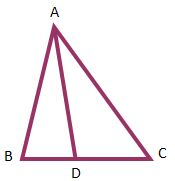#### Question 3 3. What's the value of sec2 (θ) given that the equation below is true?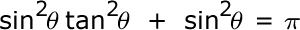#### Question 4 4. Which pair of angles are alternate interior angles?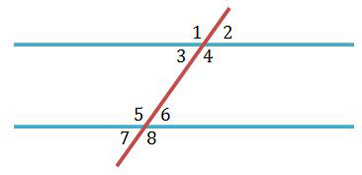#### Question 6 6. If AB and CB are tangent segments, what is the length of CB?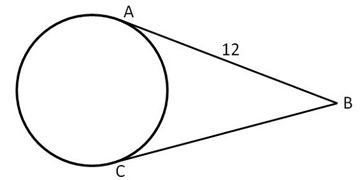#### Question 7 7. In the picture below, if arc CE is 172 degrees and arc BD is 80 degrees, what is the measure of angle CAE?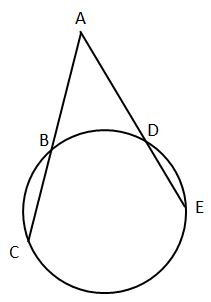#### Question 8 8. In the pictured triangle, which of the following lines is an altitude?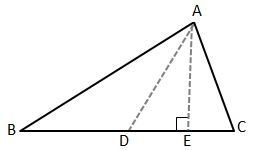#### Question 9 9. Which angle is the alternate exterior angle to angle 2?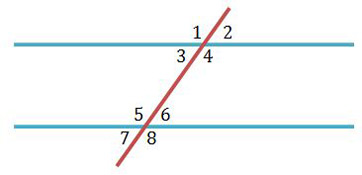#### Question 15 15. In the pictured triangle, ∠A is 137 degrees and ∠B is 28 degrees. If side b is 71 units long, approximately how long is side a?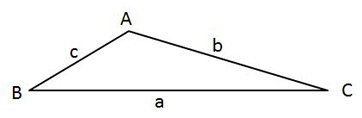## Are you a student or a teacher?

#### Geometry: High School Final Free Practice Test Instructions

Choose your answer to the question and click 'Continue' to see how you did. Then click 'Next Question' to answer the next question. When you have completed the free practice test, click 'View Results' to see your results. Good luck!

Support